## Powers and Exponents

An exponent is a positive or negative number placed above and to the right of a quantity. It expresses the power to which the quantity is to be raised or lowered. In 43, 3 is the exponent and 4 is called the base. It shows that 4 is to be used as a factor three times. 4 × 4 × 4 (multiplied by itself twice). 43 is read as four to the third power (or four cubed).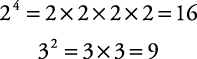Remember that x 1 = x and x 0 = 1 when x is any number (other than 0).If the exponent is negative, such as 3–2, then the base can be dropped under the number 1 in a fraction and the exponent made positive. An alternative method is to take the reciprocal of the base and change the exponent to a positive value.

##### Example 1

Simplify the following by changing the exponent from a negative value to a positive value and then evaluate the expression.#### Squares and cubes

Two specific types of powers should be noted, squares and cubes. To square a number, just multiply it by itself (the exponent would be 2). For example, 6 squared (written 62) is 6 × 6, or 36. 36 is called a perfect square (the square of a whole number). Following is a list of the first twelve perfect squares:To cube a number, just multiply it by itself twice (the exponent would be 3). For example, 5 cubed (written 53) is 5 × 5 × 5, or 125. 125 is called a perfect cube (the cube of a whole number). Following is a list of the first twelve perfect cubes.#### Operations with powers and exponents

To multiply two numbers with exponents, if the base numbers are the same, simply keep the base number and add the exponents.

##### Example 2

Multiply the following, leaving the answers with exponents.

1.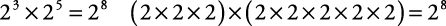2.To divide two numbers with exponents, if the base numbers are the same, simply keep the base number and subtract the second exponent from the first, or the exponent of the denominator from the exponent of the numerator.

##### Example 3

Divide the following, leaving the answers with exponents.

1.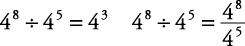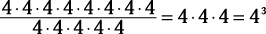2.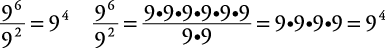To multiply or divide numbers with exponents, if the base numbers are different, you must simplify each number with an exponent first and then perform the operation.

##### Example 4

Simplify and perform the operation indicated.

1. 32 × 22 = 9 × 4 = 36

2.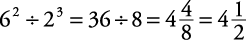(Some shortcuts are possible.)

To add or subtract numbers with exponents, whether the base numbers are the same or different, you must simplify each number with an exponent first and then perform the indicated operation.

##### Example 5

Simplify and perform the operation indicated.

1. 32 – 23 = 9 – 8 = 1

2. 43 + 32 = 64 + 9 = 73

If a number with an exponent is raised to another power (42)3, simply keep the original base number and multiply the exponents.

##### Example 6

Multiply and leave the answers with exponents.

1.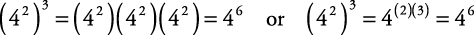2.